# New to Qlik Sense

If you’re new to Qlik Sense, start with this Discussion Board and get up-to-speed quickly.

Announcements
QlikWorld 2023, a live, in-person thrill ride. Save \$300 before February 6: REGISTER NOW!
cancel
Showing results for
Did you mean:Contributor III

## Formatting

Hi Gurus,

I have created the below expression and its works as expected:

sum(aggr(sum(aggr(Count(distinct[Emp]),[Date],[Dep],[Com])),[We]))/
count(total<[We]>distinct[Date])

I get the value 1278,000,0

but i need this value to be 1278

How can I get this please.

Many Thanks,

Kumar

Labels (8)

• ### Visualization

1 Solution

Accepted Solutions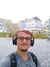Specialist

Hello,

Can you please share with us a screenshot of how it looks like on your side? It will allow us to understand where exactly this value is presented and how else can we proceed further. My current hypothesis is that additional formatting is applied on the response.

For example:

1. If the formatting is applied on measure itself.
2. If the formatting is applied on Master item measure.
3. etc.

Try expanding the measure and check the con figuration there: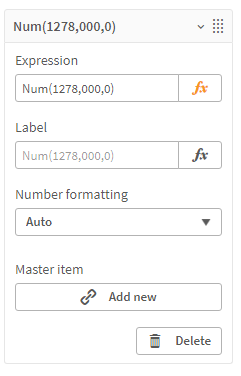For example on my side, it is set to auto and thus the number is "1278". If I set it to custom then my number changes to "1,278.00". So on your side most probably this is what causing the number to appear as it does, regardless of the formatting you pass in the expression.

Help users find answers! Don't forget to mark a solution that worked for you! 🙂
8 RepliesSpecialist

Hello,

Have you tried wrapping it into Num() function? Like Num(EXPRESSION) ?

For example:

=Num(sum(aggr(sum(aggr(Count(distinct[Emp]),[Date],[Dep],[Com])),[We]))/
count(total<[We]>distinct[Date]))

On my side the output is: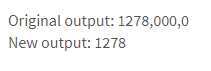NOTE: You can also take a look at the official documentation page , where it shows how to use custom formatting with the function, to choose how the output number will look like.

---

I hope that this information was helpful. In case I have misunderstood the use case scenario, please elaborate in details by providing additional information. However, if it has helped you resolve the issue, addressed your concerns or at least pointed you in the right direction, please mark it as Accepted Solution to give further visibility to other community members.

Help users find answers! Don't forget to mark a solution that worked for you! 🙂Contributor III
Author

Hi Andrei,

I tried Num at my end and it still show the same value 1278,000,0

I used this:
=Num(sum(aggr(sum(aggr(Count(distinct[Emp]),[Date],[Dep],[Com])),[We]))/
count(total<[We]>distinct[Date]))

Thanks,

KumarSpecialist

Hello,

Can you please share with us a screenshot of how it looks like on your side? It will allow us to understand where exactly this value is presented and how else can we proceed further. My current hypothesis is that additional formatting is applied on the response.

For example:

1. If the formatting is applied on measure itself.
2. If the formatting is applied on Master item measure.
3. etc.

Try expanding the measure and check the con figuration there:For example on my side, it is set to auto and thus the number is "1278". If I set it to custom then my number changes to "1,278.00". So on your side most probably this is what causing the number to appear as it does, regardless of the formatting you pass in the expression.

Help users find answers! Don't forget to mark a solution that worked for you! 🙂Contributor III
Author

Hi Andrei,

Apologies ! its my bad I have selected Number at my end and once I changed to Auto it showing the correct value 1278.

Many Thanks,

KumarCreator

Olá,  @pjpkumar26

Você pode tentar usar essa expressão

if(soma(aggr(soma(aggr(Count(distinct[Emp]),[Data],[Dep],[Com])),[We]))

/

num( soma(aggr(soma(aggr(Contagem(distinta[Emp]),[Data],[Dep],[Com])),[We]))

/
count(total<[ Nós]>distinto[Data]) )/1E6,'#,00 M')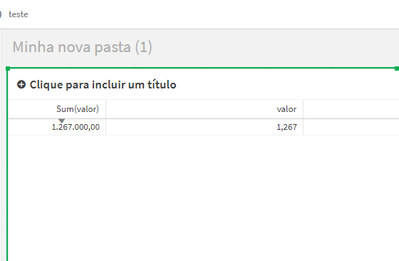Caso tenha ajudado a marcar como solução e deixe um like

Grato!Creator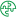Contribuinte III

Olá,  @pjpkumar26

if(soma(aggr(soma(aggr(Count(distinct[Emp]),[Data],[Dep],[Com])),[We]))

/

num( soma(aggr(soma(aggr(Contagem(distinta[Emp]),[Data],[Dep],[Com])),[We]))

/
count(total<[ Nós]>distinto[Data]) )/1E6,'#,00 M')

Caso tenha ajudado a marcar como solução e deixe um like

Grato!Contributor III
Author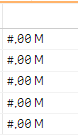I get this when i use the above syntax - can't see the value

RegardsCreator

Olá, @pjpkumar26

Veja a a essa sintaxe

if(

sum(aggr(sum(aggr(Count(distinct[Emp]),[Data],[Dep],[Com])),[We]))
/

num(sum(aggr(sum(aggr(count(distinct[Emp]),[Data],[Dep],[Com])),[We]))

/
count(total<[ Nós]>distinct[Data])/1E6,'#,000 M')
)

Aqui utilizei um teste e está funcionando bem.Tags
Community Browser